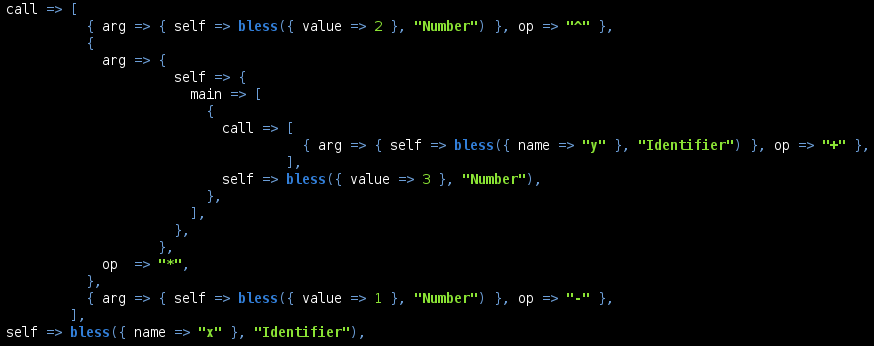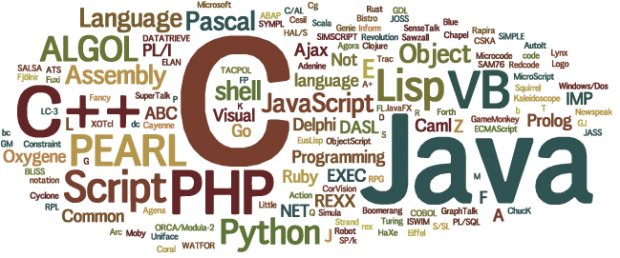## Posts

Showing posts from 2015

### Parsing and code generationIn this post we're going to take a close look at how to put together a basic parser and how to generate real code from the Abstract Syntax Tree (AST). # Overview A couple of posts ago, we talked about the basics behind the creation of a programming language , but we didn't look at any real code how to actually do that. This time, we're going to get our hands dirty and write a real parser and a code generator for a very small language subset. # The parser  For simplicity, our parser will be able to understand only variable declarations, numbers, arithmetic operators, nested expressions and the say  keyword which will print something to the screen. The first thing before writing a parser, it's the design of the AST that it should produce. The AST have a recursive definition with sub-branches that resembles the top of the tree. A very simple design for the AST, is the following: main the top of the AST self an object or an expression call a

### Finding similar imagesIn this post we're going to implement a simple algorithm which finds images that look similar to each other. # Overview In order to find images that look similar, we first need to normalize them to a simpler form and compute a fingerprint for each image. The fingerprint is a binary sequence of ones and zeros and it's created from the average color of the image and the average color of each pixel. In order to make the process faster and more flexible (and also to have fingerprints of the same length) the image is first resized to a fixed resolution, which defaults to 64x64. # Algorithm This is the algorithm which creates the fingerprint of an image: averages = [ ] for y in range ( height ) { for x in range ( width ) { rgb = img . get_pixel ( x , y ) averages . append ( sum ( rgb ) / len ( rgb ) ) } } avg = sum ( averages ) / len ( averages ) fingerprint = averages . map { | v | v < avg ? 1 : 0 } It

### Creating a programming languageIn this post we're going to look over the main things required in designing and implementing a programming language. # Overview Before writing any code and before designing any language construct, we need to think about the core of the language that we want to create. The core of the language is the most important part which defines the language itself. We also have to choose a  programming paradigm  for our language from the following list: imperative declarative functional object-oriented procedural logic symbolic A language can have more than one paradigm. For example, it can be imperative and object-oriented at the same time. These are called multi-paradigm languages. After these two criteria are met, we can start thinking about a syntax for our language and begin carefully to design language constructs and implement them. In order to implement our programming language, we need to write a parser which creates an abstract syntax tree (AST) from our s

### Semiprime equationizationPrime numbers play an important role in cryptography due to the fact that it's hard to factorize a semiprime into its original two factors. A while ago, RSA put prizes on large semiprimes and challenged the public to crack them. Not surprisingly, most of the numbers remained unfactored until this day. The entire security system is based on the fact that we don't have a truly efficient factorization algorithm. To give you an example, using the most efficient known algorithm ( GNFS ), it took 5 months to factorize the RSA-640 number using 80 AMD Opteron CPUs, clocked at 2.2 GHz. But there is something special about the RSA semiprimes; all of them are the product of two prime numbers which have about the same length, with a deviation of ± 1 in some cases. This is a very valuable piece of information, because we can test only a restricted amount of prime numbers that are in the range we are interested in. For example, RSA-100  is a 100-digit number and is the product o